# General organic chemistry Questions and Answers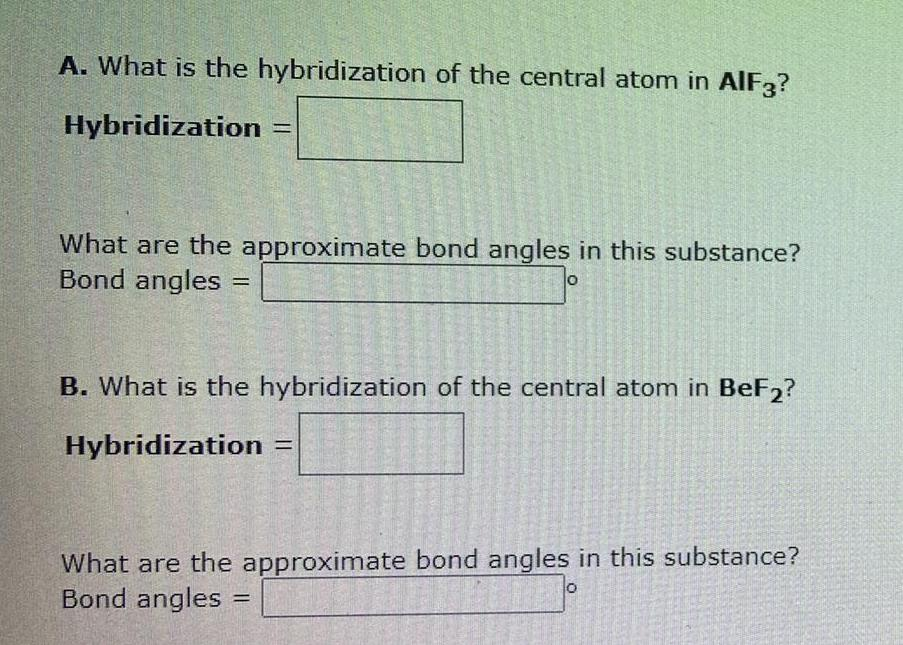Organic Chemistry
General organic chemistry
A. What is the hybridization of the central atom in AlF3? Hybridization What are the approximate bond angles in this substance? Bond angles B. What is the hybridization of the central atom in BeF2? Hybridization What are the approximate bond angles in this substance? Bond angles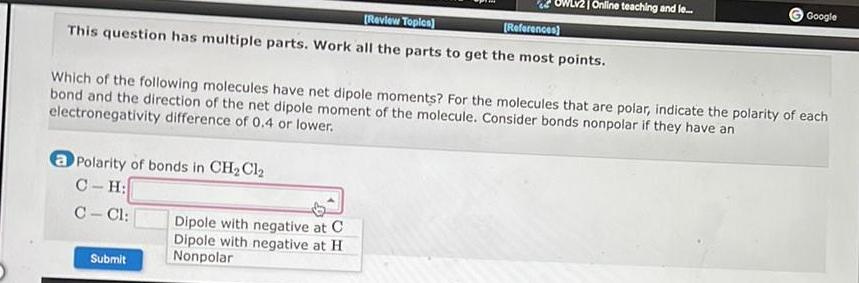Organic Chemistry
General organic chemistry
This question has multiple parts. Work all the parts to get the most points. a Polarity of bonds in CH₂Cl₂ C-H: C-Cl: Which of the following molecules have net dipole moments? For the molecules that are polar, indicate the polarity of each bond and the direction of the net dipole moment of the molecule. Consider bonds nonpolar if they have an electronegativity difference of 0.4 or lower. Dipole with negative at C Dipole with negative at H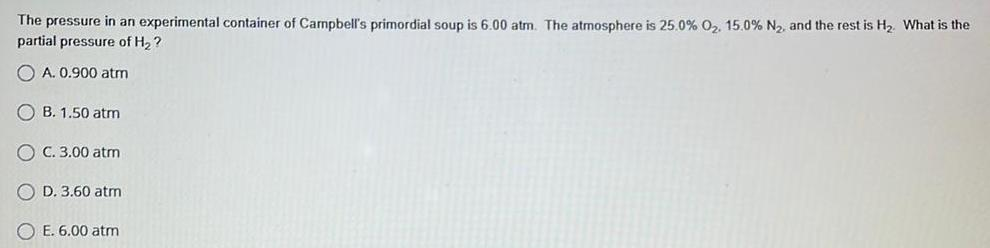Organic Chemistry
General organic chemistry
The pressure in an experimental container of Campbell's primordial soup is 6.00 atm. The atmosphere is 25.0% 0₂. 15.0 % N₂, and the rest is H₂. What is the partial pressure of H₂? A. 0.900 atm B. 1.50 atm C. 3.00 atm D. 3.60 atm E. 6.00 atm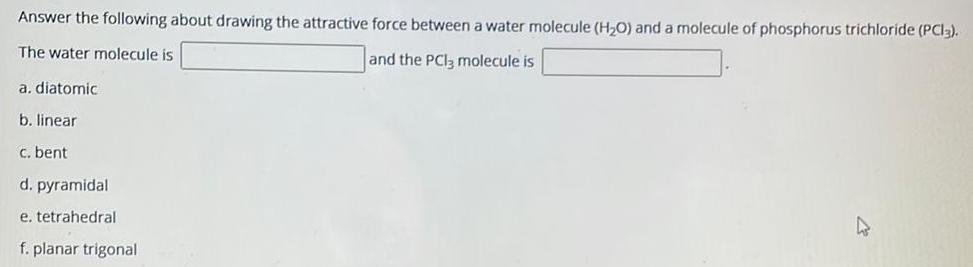Organic Chemistry
General organic chemistry
Answer the following about drawing the attractive force between a water molecule (H₂O) and a molecule of phosphorus trichloride (PCI). The water molecule is and the PCI3 molecule is a. diatomic b. linear c. bent d. pyramidal e. tetrahedral f. planar trigonal 4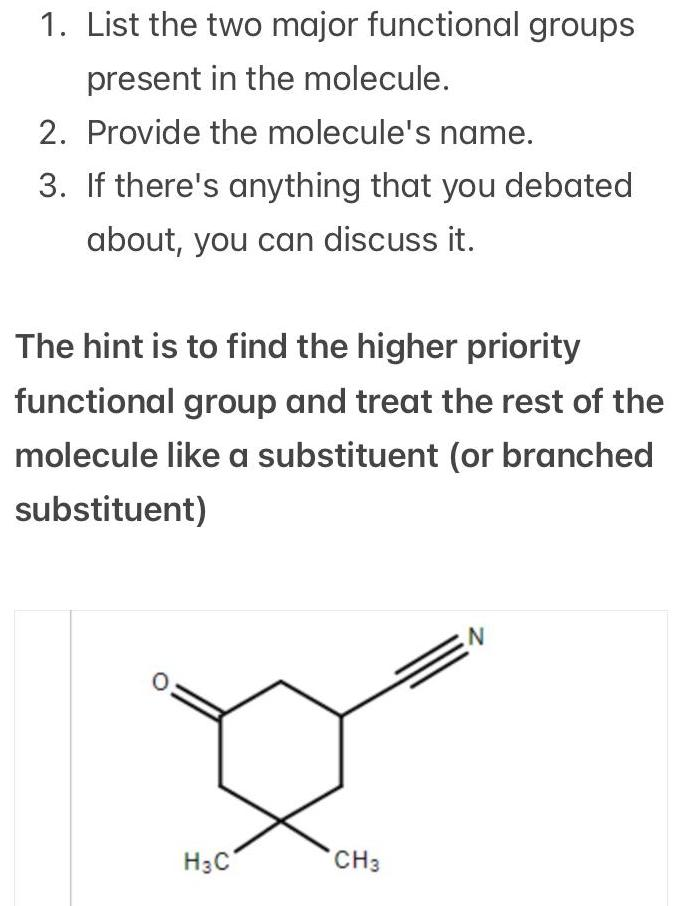Organic Chemistry
General organic chemistry
1. List the two major functional groups present in the molecule. 2. Provide the molecule's name. 3. If there's anything that you debated about, you can discuss it. The hint is to find the higher priority functional group and treat the rest of the molecule like a substituent (or branched substituent)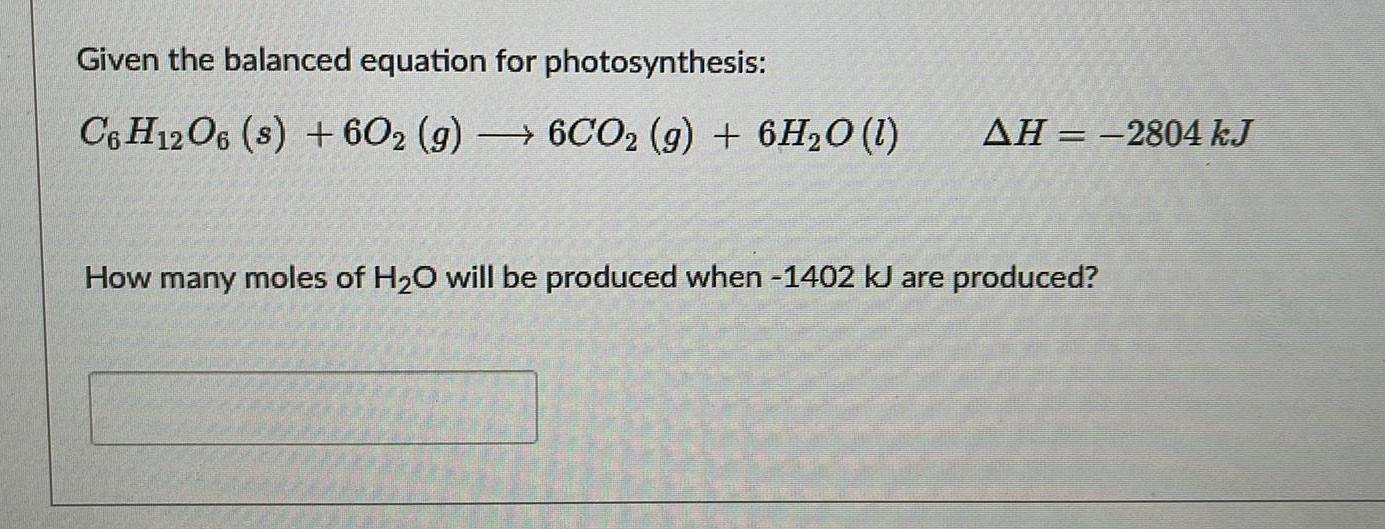Organic Chemistry
General organic chemistry
Given the balanced equation for photosynthesis: C6H12O6 (s) + 602 (g) 6CO2 (g) + 6H2O (1) AH-2804 kJ How many moles of H₂O will be produced when -1402 kJ are produced?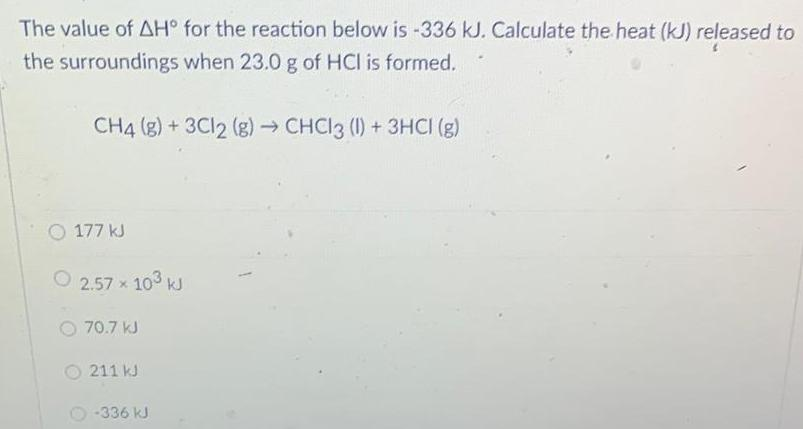Organic Chemistry
General organic chemistry
The value of AH° for the reaction below is -336 kJ. Calculate the heat (kJ) released to the surroundings when 23.0 g of HCI is formed. CH4 (g) + 3Cl2 (g) → CHCl3 (1) + 3HCI (g) 177 kJ 2.57 × 10³ kJ 70.7 kJ 211 kJ -336 kJ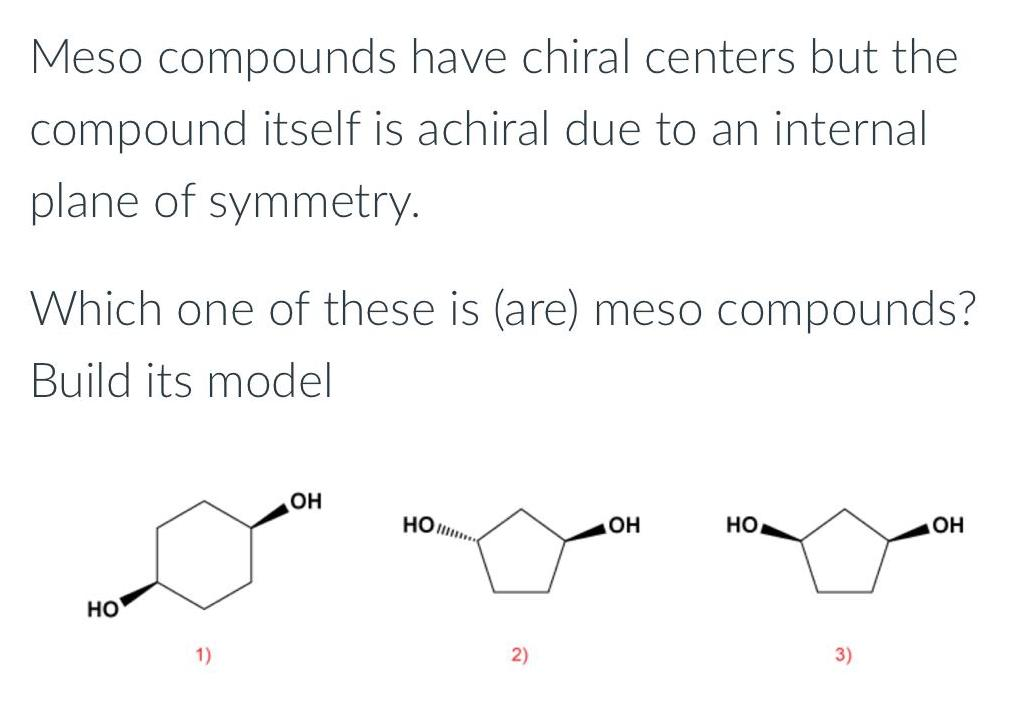Organic Chemistry
General organic chemistry
Meso compounds have chiral centers but the compound itself is achiral due to an internal plane of symmetry. Which one of these is (are) meso compounds? Build its model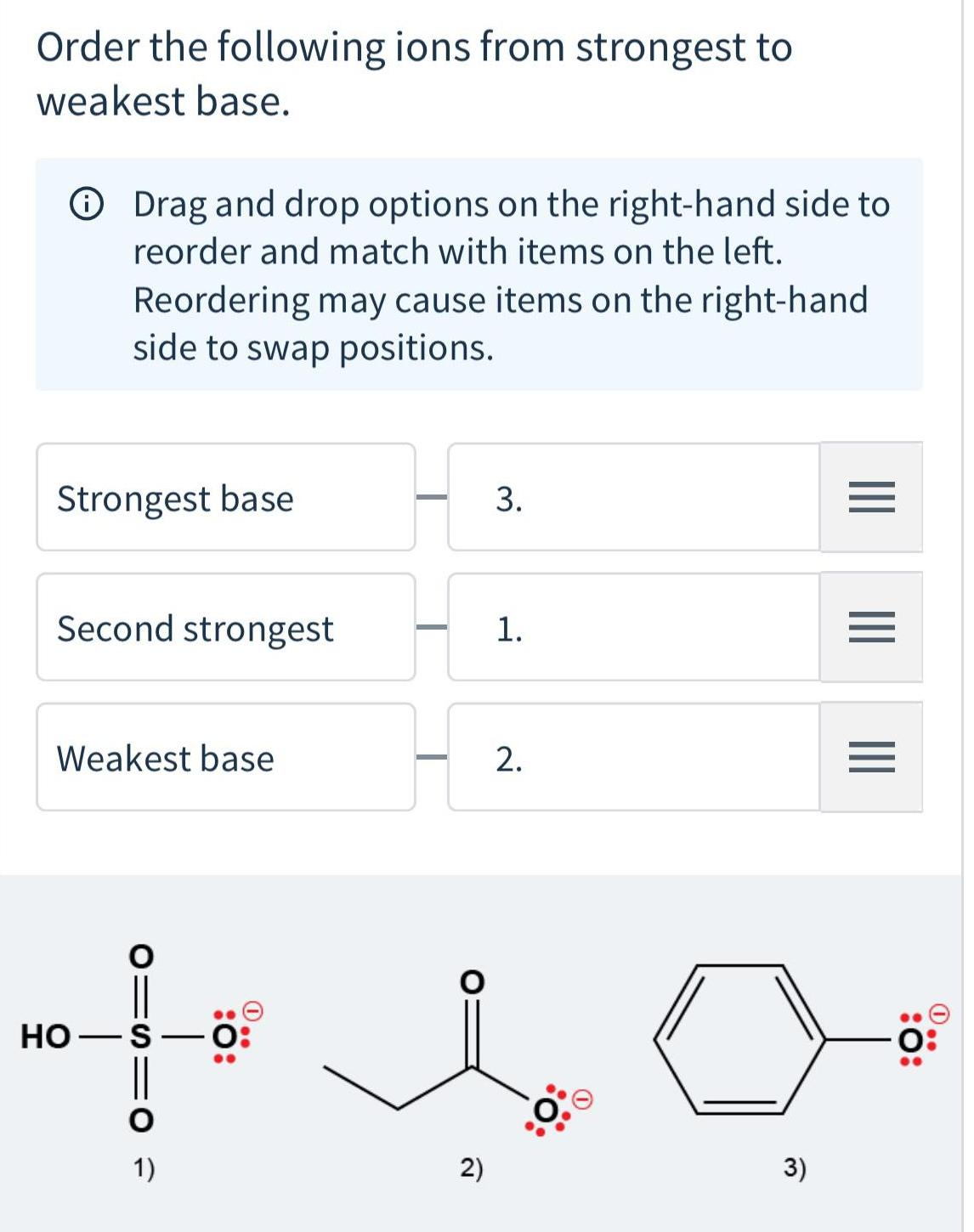Organic Chemistry
General organic chemistry
Order the following ions from strongest to weakest base. Strongest base Drag and drop options on the right-hand side to reorder and match with items on the left. Reordering may cause items on the right-hand side to swap positions. Second strongest Weakest base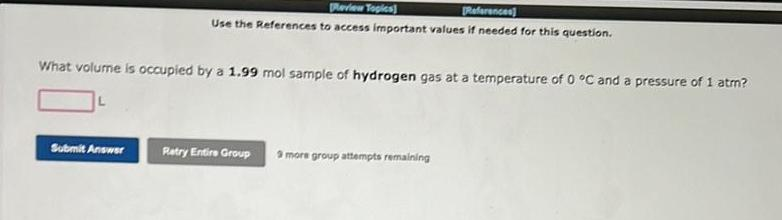Organic Chemistry
General organic chemistry
Use the References to access important values if needed for this question. What volume is occupied by a 1.99 mol sample of hydrogen gas at a temperature of 0 °C and a pressure of 1 atm?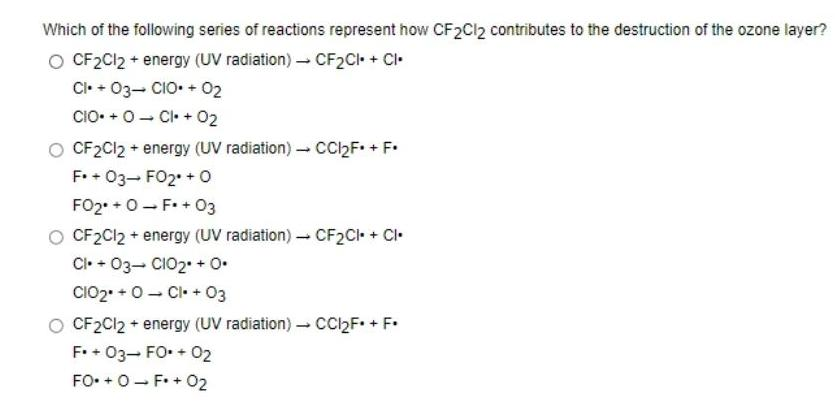Organic Chemistry
General organic chemistry
Which of the following series of reactions represent how CF2Cl2 contributes to the destruction of the ozone layer? CF2Cl2 + energy (UV radiation)→ CF₂CI+ + Cl. CI. + 03- CI+ 02 CI+ CI+ 02 CF2Cl2 + energy (UV radiation)→ CCl₂F* + F. F. + 03- FO2 + O FO₂+OF+03 CF2Cl2 + energy (UV radiation) → CF2CI+ CI. Cl. + 03- CIO2 + 0. CIO₂ + CI. + 03 CF2Cl2 + energy (UV radiation)→ CCI₂F. + F. F. + 03- FO. + 02 FO+OF+ 02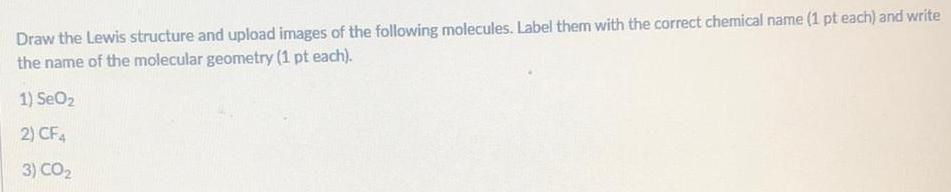Organic Chemistry
General organic chemistry
Draw the Lewis structure and upload images of the following molecules. Label them with the correct chemical name (1 pt each) and write the name of the molecular geometry (1 pt each). 1) SeO₂ 2) CF4 3) CO₂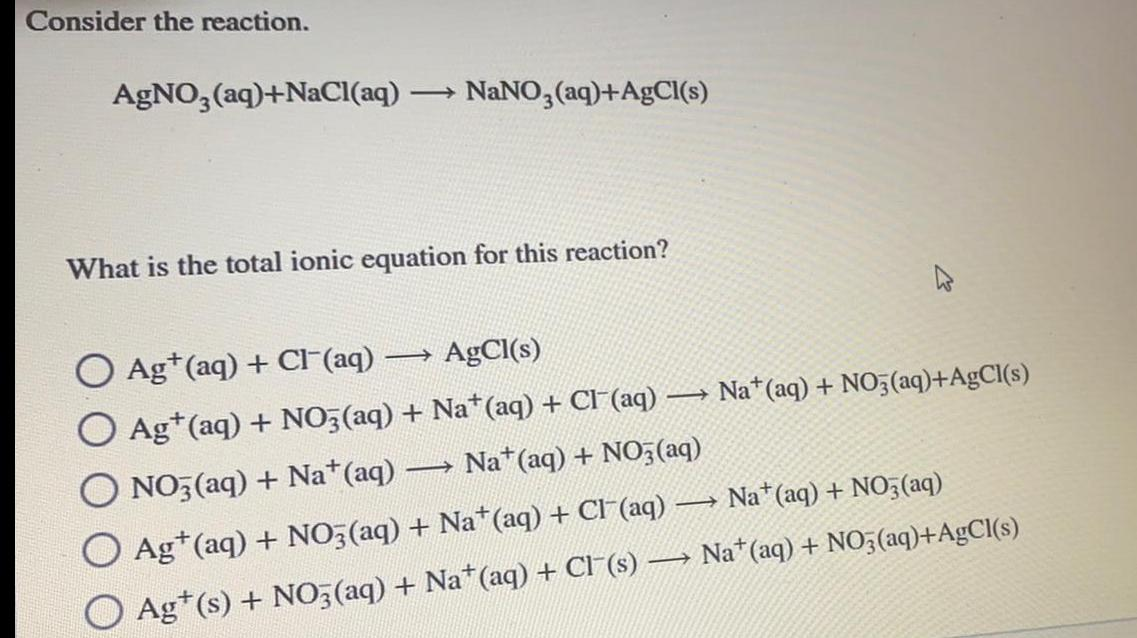Organic Chemistry
General organic chemistry
Consider the reaction. AgNO3(aq)+NaCl(aq) → NaNO3(aq)+AgCl(s) What is the total ionic equation for this reaction?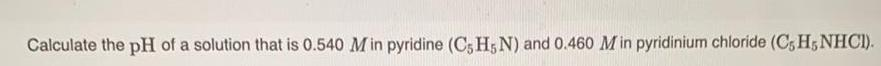Organic Chemistry
General organic chemistry
Calculate the pH of a solution that is 0.540 Min pyridine (C5 H5N) and 0.460 M in pyridinium chloride (C5 H5NHCI).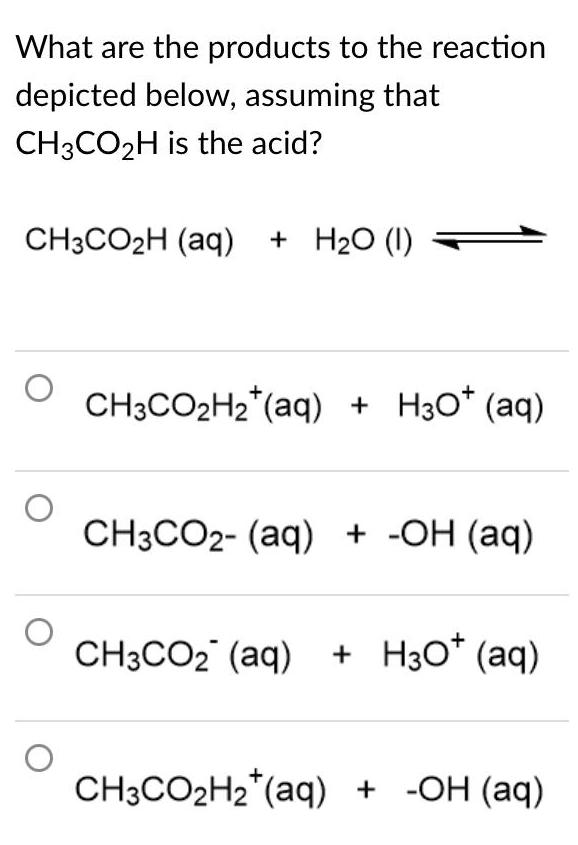Organic Chemistry
General organic chemistry
What are the products to the reaction depicted below, assuming that CH3CO₂H is the acid? CH3CO₂H (aq) + H₂O (1) CH3CO₂H₂ (aq) + H3O+ (aq) CH3CO2- (aq) + -OH (aq) CH3CO₂ (aq) + H3O+ (aq) CH3CO₂H₂(aq) + -OH (aq)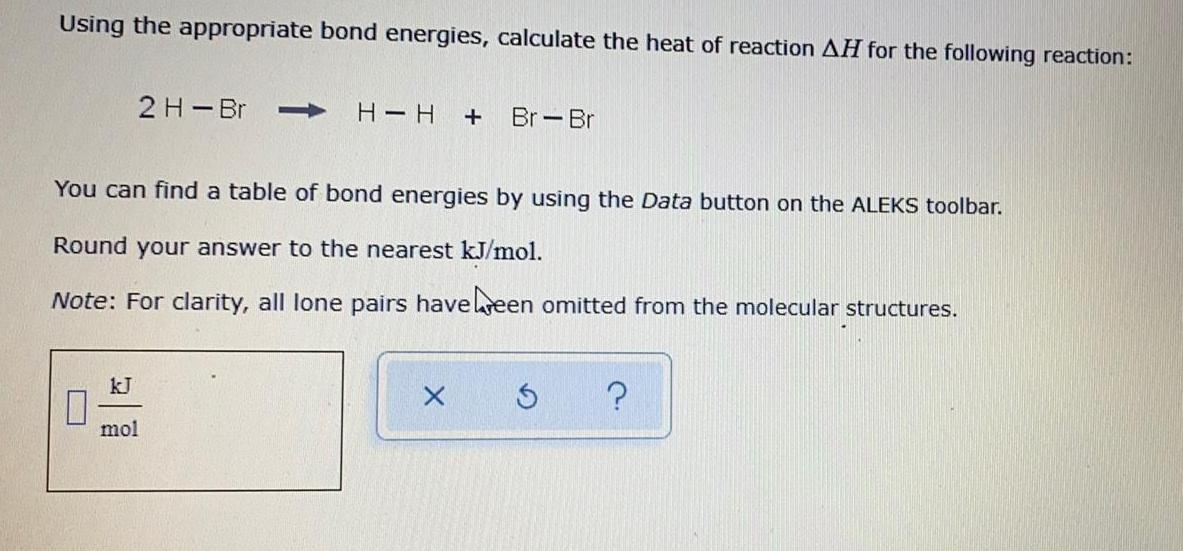Organic Chemistry
General organic chemistry
Using the appropriate bond energies, calculate the heat of reaction AH for the following reaction: 2 H-Br----> H-H + Br-Br You can find a table of bond energies by using the Data button on the ALEKS toolbar. Round your answer to the nearest kJ/mol. Note: For clarity, all lone pairs have been omitted from the molecular structures.